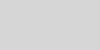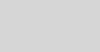# New Tutorials: Learn the Basic Concepts of Quantum ComputingMariia Mykhailova

This post is part of the Q# Advent Calendar 2019. Check out the calendar for more great posts!

Let me continue the topic of teaching and learning quantum computing that I touched upon yesterday and share with you the project done by my summer intern Artem Astapchuk – a set of tutorials that introduce the most basic concepts used in quantum computing.

### How easy is the easiest kata?

The Quantum Katas start with the BasicGates kata – a set of exercises that ask the learner to identify the quantum gate that will perform the described transformation of a qubit state. It seems like a very easy thing to do – how hard can it be to look up a list of quantum gates and match one of them to the task?

Turns out, it is pretty hard for somebody who sees the quantum computing notation for the first time. Even the easiest task that asks to apply the Pauli X gate relies on a lot of implied knowledge. What is a qubit and its state? What are these weird symbols around 0 and 1? What kind of tools do you use to change the state of the qubit? And how do you express all this in Q#?

### Let’s fix this!

Historically the Katas sent the learner to find an external source for learning the basic concepts and notations used in quantum computing. However, eventually it started to make more sense to create a set of tutorials that would teach the learner everything they need to start solving the first katas in one place and using one style.

Well, here they are! Two tutorials that cover the math necessary for working with quantum computing – complex arithmetic and linear algebra – are written in Python, and the four that introduce the quantum computing concepts – qubits and superposition, multi-qubit systems and entanglement, and single- and multi-qubit gates – are implemented in Q#.We tried to steer away from too much theory and to give just the definitions and the tools necessary for getting started (yes, qubit states are described using Hilbert spaces; no, you don’t need to know this to start learning). Instead, we focused on making the learning process as hands-on as possible, using demos and programming exercises to help learner internalize the new concepts and ideas by applying them to solving simple tasks.

For example, the definition of the imaginary unit i is immediately followed by a programming task that asks one to calculate the n-th power of i iⁿ; the introduction of the qubit states is followed by a demo that shows how to examine and interpret the qubit state in Q# using the DumpMachine function, and so on.### Next steps

If you’re looking to get started with quantum computing, give these tutorials a try and let us know what you think!

Meanwhile, we’ll keep working on bringing you more tutorials. Next up: measurements!